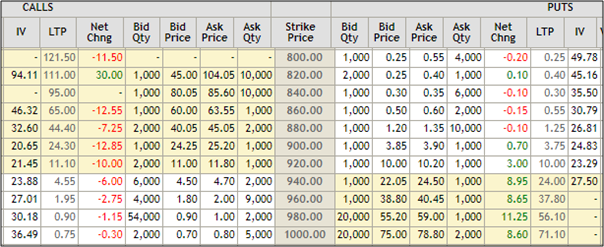You must have intuitively noticed that whenever the volatility in the market goes up, the value of the option also goes up. Now that surely sounds contrary right? After all, we always believed that value is inversely related to risk. Then how does the value of the option go up when the volatility increases. Before understanding the importance of volatility in options, it is essential to understand the concept of volatility first. Subsequently, we shall also delve deeper into the role of volatility in options calculation as well as the volatility options strategies that can be profitably employed.

But first, what do we understand by volatility? Volatility is the probability that the price of the stock will vary substantially from the average price (mean price). Volatility is typically measured by a statistical measure called the standard deviation which measures to what extent the price of a stock varies from the mean or the average price. Therefore, volatility is also a measure of risk. In the Indian market, volatility risk is depicted using the popular Volatility Index (VIX), which is calculated by the NSE on a real time basis. Having understood the concept of volatility, let us see how the volatility impacts the option values.

Importance of volatility in options valuation..
To understand the role of volatility, let us take the live option chain of Reliance Industries for the February 2018 options contracts. We shall consider calls and puts in this chain.Source: NSE

At the time of capturing this option chain, the spot price of Reliance Industries was ruling at Rs.920. While call options are captured on the left side of the table, put options are captured on the right side of the table. Let us take the case of the 940 RIL Call option and the 920 RIL Call option for the Feb 2018 contract. The RIL 920 call is an at-the-money (ATM) call, which has an intrinsic value of zero. However, it is quoting in the market at Rs.11.80, which is entirely the time value of the option. Similarly, the 940 RIL call option is OTM and has no intrinsic value and hence the entire premium of Rs.4.70 is the time value of the option. Let us now turn to the put options. The ITM 940 RIL put has an intrinsic value of Rs.20 but is quoting at Rs.24.50 with Rs.4.50 representing the time value of the option. In case of the OTM 900 RIL put option, the intrinsic value is zero so that entire premium of Rs.3.90 represents time value of option.

Why are we focusing so much on the time value of the option? Remember, the intrinsic value of the option represents the reality and the time value represents the expectations of value. As volatility increases, there is expectation of greater movement in prices and therefore the time value of the option increases. This happens even when the stock price may have hardly moved. That is why volatility becomes important as it is one of the key factors that drive the time value of the option, and therefore the premium commanded by the option.

But why do calls and puts benefit from higher volatility?
While volatility is risk for equities, it actually becomes a source of returns in case of options. Let us look at options values of calls and puts in a hypothetical example under situations of lower and higher volatility. We assume that the spot price of RIL is Rs.920/- We also assume that the 940 Call and 900 Put are having a premium value of Rs.4/-..

 RIL 940 Call Option (Feb 2018 expiry) RIL 900 Put Option (Feb 2018 expiry) Particulars Intrin / Time Total Value Particulars Intrin / Time Total  Value Vol (+5 %) - 966 26 / 10 36 Vol (+5 %) - 966 00 / 01 01 Vol (-5 %) - 874 00 / 01 01 Vol (-5 %) - 874 26 / 10 36 Vol (+10 %) - 1012 72 / 20 92 Vol (+10 %) - 1012 00 / 00 00 Vol (-10 %) - 828 00 / 00 00 Vol (-10 %) - 828 72 / 20 92

The above table depicts that as the volatility increases, the time value of the options is also increasing with the volatility. For a call option, volatility means the stock price could go up sharply or go down sharply. When the stock goes down, the call options will not be exercised and the loss will be limited to the premium. On the upside the benefit of volatility is entirely the option buyer 's.

In case of put option, the buyer will let the option expire in case of upside volatility and profit in times of downside volatility. Volatility makes calls and puts more valuable even when prices do not really move. That is why volatility is so important.

Using volatile strategies via options..
Most traders are quite clear what to do with options when the market is expected to go up or go down. But what do you do when you are not sure of the direction of the market but are quite clear that volatility will increase. That is when you can play on the time value and that is where strategies like long straddles and strangles can be useful. The beauty of volatility is that it benefits the buyers of calls and puts and both can benefit from higher volatility irrespective of actual price movement.